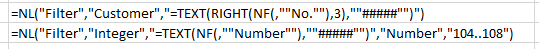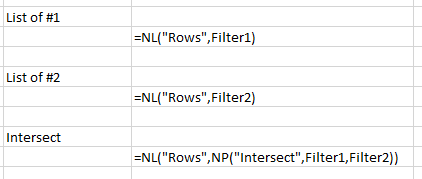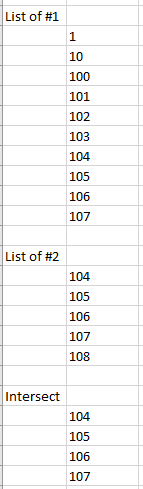I have a filter that returns a list of numbers:

=NL("Filter","Estimation Entry","=VALUE(NF(,""Bill Item""))","Job No.","1234")

(The VALUE part is because they are stored as text values with leading zeros, don't ask).

Due to the problem with the data being stored as text, I then want to do an intersect on it with a list of numbers to provide some filtering:

=NL("Filter","Integer","Number","Number","0..14")

If I do a union of the 2 I get the expected results - a combination of the 2 (out of order but that's another issue)

=NL("Rows",NP("Union",Filter1,Filter2))

But if I do an intersect I get no values returned. I know there are values that appear in both sets.

=NL("Rows",NP("Intersect",Filter1,Filter2))

(Actually my problem is slightly more complex as I've got an extra union in there with another table, however - in my attempts to break down the problem I've found this simpler scenario that doesn't work).

Anyone got any ideas as to why it isn't working?

•Harry Lewis

Hello Teresa -

Yep, I have an idea.

At issue here is that your Filter2 contains integers.

Excel's VALUE() function returns Double-Precision values (not integers) and, thus, cannot compare the two successfully.

Think of it this way.  For the text "104", we can create an Integer 104, while Excel's VALUE function creates the number 104.000

To Excel 104.000 and 104 do not intersect.

So, what can we do about it?

Here's my idea...

Treat EVERYTHING as though it were text (this gives us much more control over the formatting).

So, I created a filter from my data (I used the last three digits of my customer numbers) and another filter using the Integer table:As you can see, I use Excel TEXT() function to put both lists in the identical format.

I then wrote three NL(Rows) functions:  one to list my first filter, one to list the second filter, and the last to show the intersect:When I run my report:I get the true intersect between my two filters.

I hope that helps.

•Teresa Roberts

Hi,

That works great. More of an excel question than jet reports but one of the values returned is "0". However, that's being returned as a blank row rather than the number 0, which my filters using that cell don't like.

•Teresa Roberts

Using INT instead of TEXT worked.